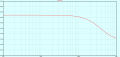# Calculating The Input Impedance Of A Class AB Amp

Joined Jun 16, 2023
128
I know that the input impedance of the following amp is approximately 15K.
I would like to calculate the impedance mathematically but I am not sure
what the ac equivalent circuit looks like. I realize that connections to VCC
are considered to be at AC ground and capacitors are considered to be
shorts. Then I am lost.

#### BobTPH

Joined Jun 5, 2013
8,068
Capacitors have an impedance that is frequency dependent. The input coupling capacitor should have an impedance that is low compared to the 15K for the lowest frequency you are interested in.

Joined Jun 16, 2023
128
Capacitors have an impedance that is frequency dependent. The input coupling capacitor should have an impedance that is low compared to the 15K for the lowest frequency you are interested in.
The impedance of a 10μF capacitor at 20Hz is 796Ω << 15KΩ.

#### Audioguru again

Joined Oct 21, 2019
6,432
To find the average input impedance I simply increased the input resistor until the p-p average output level was halved.
But I think this simple circuit has an unsymmetrical input impedance then positive-going input signals (that cause the Q1 transistor base current to increase) have a low impedance and negative-going input signals have a much higher impedance.

#### Attachments

Joined Jun 16, 2023
128
To find the average input impedance I simply increased the input resistor until the p-p average output level was halved.
But I think this simple circuit has an unsymmetrical input impedance then positive-going input signals (that cause the Q1 transistor base current to increase) have a low impedance and negative-going input signals have a much higher impedance.
How does that indicate the input impedance?

#### Audioguru again

Joined Oct 21, 2019
6,432
The actual input impedance of an amplifier is used for the input coupling capacitor calculation. The actual input impedance of the amplifier does not matter since the impedance of the signal source must be much lower.

I assumed an input impedance of 19k ohms on the post of my latest simulation.

Joined Jun 16, 2023
128
The actual input impedance of an amplifier is used for the input coupling capacitor calculation. The actual input impedance of the amplifier does not matter since the impedance of the signal source must be much lower.

I assumed an input impedance of 19k ohms on the post of my latest simulation.
Got it. Since the series input resistor is 15K that would mean that 4K is coming from somewhere else
in the circuit.

Last edited:

#### Audioguru again

Joined Oct 21, 2019
6,432
"Somewhere else" is on the datasheet of the 2N3904 transistor.

#### Attachments

Joined Jun 16, 2023
128
"Somewhere else" is on the datasheet of the 2N3904 transistor.
Hi AG:

This is my circuit sim that is intended to display the input impedance.
See attached.

My input impedance calculation:
r'e = 25mV / IE = 25mV/9mA = 2.778Ω
assume βac = βdc = 300
Rin(base) = βac * r'e = 300 * 2.778 = 883.4Ω
Rin(total) = R1 + [(R2 + R3)║Rin(base)] = 15K + [(360KΩ + 470Ω)║883.4Ω] = 15.831KΩ versus 15.532KΩ in sim

RS

Last edited:

#### Audioguru again

Joined Oct 21, 2019
6,432
Why do you show a simulation of the frequency response but with the output level much too high which produces severe clipping? You cannot calculate the input impedance with the output clipping. Also, the collector current in Q1 is less than 10mA, in my post #4 the collector current is only 4.7mA. Then the transistor input impedance is much higher than yours.
I showed voltage levels, not dBs.

Why are the parts and texts on your simulation so far apart that they are tiny and cannot be seen?

Joined Jun 16, 2023
128
Why do you show a simulation of the frequency response but with the output level much too high which produces severe clipping? You cannot calculate the input impedance with the output clipping. Also, the collector current in Q1 is less than 10mA, in my post #4 the collector current is only 4.7mA. Then the transistor input impedance is much higher than yours.
I showed voltage levels, not dBs.

Why are the parts and texts on your simulation so far apart that they are tiny and cannot be seen?
This is what my sim shows. I don't know what you are looking at.#### Audioguru again

Joined Oct 21, 2019
6,432
With a 1V peak input signal level, your clipping distortion is so high (maybe 30%?) that I did not measure it.

You cannot see if the waveform has clipping when you show the frequency response. Instead you must show the output signal voltage waveform like here:

#### Attachments

Joined Jun 16, 2023
128
With a 1V peak input signal level, your clipping distortion is so high (maybe 30%?) that I did not measure it.

You cannot see if the waveform has clipping when you show the frequency response. Instead you must show the output signal voltage waveform like here:
I have increased the ps to 20V.
With a 1V peak input signal level, your clipping distortion is so high (maybe 30%?) that I did not measure it.

You cannot see if the waveform has clipping when you show the frequency response. Instead you must show the output signal voltage waveform like here:
Yes, I see what you mean. I reduced the input signal to 380mV and the THD is now 1.9%. Not sure if that
would be considered to be a lot of distortion or not.

Last edited:

#### Audioguru again

Joined Oct 21, 2019
6,432
Fairly good audio amplifier ICs have distortion that is only 0.002% at 40W into 8 ohms or 0.004% distortion at 60W into 4 ohms.
Your 1.9% distortion sounds awful is far from being High Fidelity.